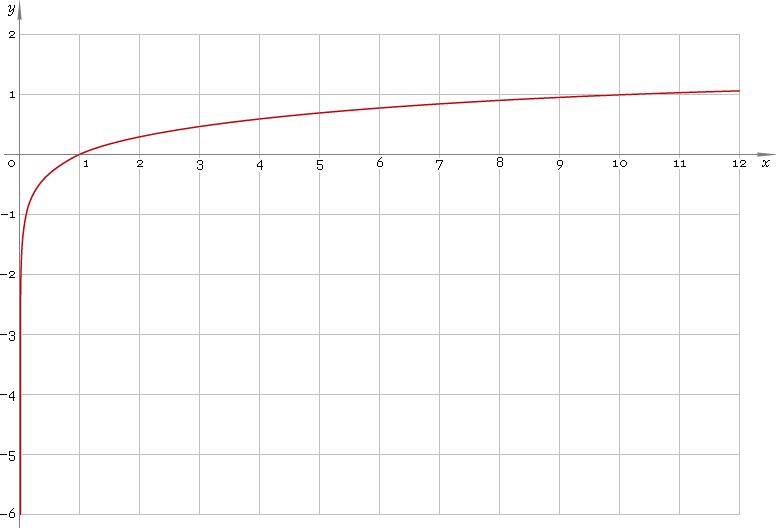The Art of Mathematics

# log or lg — decimal logarithmic function

## 1. Definition

Logarithmic function is the inverse of the 10-base exponential function

10x

## 2. Graph

Decimal logarithmic function is defined on positive part of the real axis — so its domain is (0, +∞). 0 is a singular point. Function graph is depicted below — fig. 1.Fig. 1. Graph of the decimal logarithmic function y = logx.

Function codomain is entire real axis.

## 3. Identities

By definition:

log10xx

Reciprocal argument:

log(1/x) = −logx

Product and ratio of arguments:

log(xy) = logx + logy
log(x /y) = logx − logy

Power of argument:

logxa = a logx

Base change:

logax = logx /loga
logax = logbx /logba

## 4. Derivative and indefinite integral

Decimal logarithm derivative:

log′x = 1 /(x ln10)

As well:

log′|x| = 1 /(x ln10)

Indefinite integral of the decimal logarithm:

∫ logx dx = (x lnxx) / ln10 + C

where C is an arbitrary constant.

## 5. How to use

To calculate decimal logarithm of the number:

``log(2);``

To get decimal logarithm of the complex number:

``log(2+i);``

To get decimal logarithm of the current result:

``log(rslt);``

To get decimal logarithm of the number z in calculator memory:

``log(mem[z]);``

## 6. Support

Decimal logarithm of the real argument is supported in free version of the Librow calculator.

Decimal logarithm of the complex argument is supported in professional version of the Librow calculator.